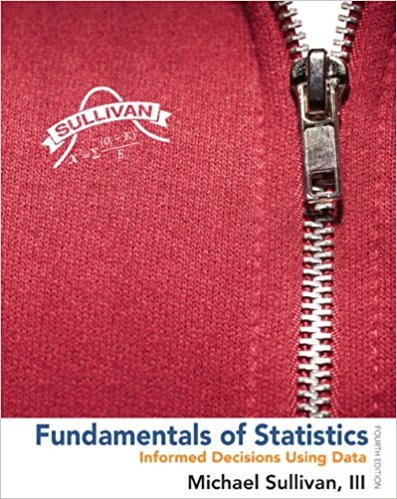×
×

# Solutions for Chapter Chapter 10.3: Fundamentals of Statistics 4th Edition## Full solutions for Fundamentals of Statistics | 4th Edition

ISBN: 9780321838704Solutions for Chapter Chapter 10.3

Solutions for Chapter Chapter 10.3
4 5 0 354 Reviews
24
0
##### ISBN: 9780321838704

Since 42 problems in chapter Chapter 10.3 have been answered, more than 269770 students have viewed full step-by-step solutions from this chapter. This expansive textbook survival guide covers the following chapters and their solutions. Chapter Chapter 10.3 includes 42 full step-by-step solutions. Fundamentals of Statistics was written by and is associated to the ISBN: 9780321838704. This textbook survival guide was created for the textbook: Fundamentals of Statistics, edition: 4.

Key Statistics Terms and definitions covered in this textbook
• 2 k p - factorial experiment

A fractional factorial experiment with k factors tested in a 2 ? p fraction with all factors tested at only two levels (settings) each

A formula used to determine the probability of the union of two (or more) events from the probabilities of the events and their intersection(s).

• Alias

In a fractional factorial experiment when certain factor effects cannot be estimated uniquely, they are said to be aliased.

• Arithmetic mean

The arithmetic mean of a set of numbers x1 , x2 ,…, xn is their sum divided by the number of observations, or ( / )1 1 n xi t n ? = . The arithmetic mean is usually denoted by x , and is often called the average

• Biased estimator

Unbiased estimator.

• Bimodal distribution.

A distribution with two modes

• Categorical data

Data consisting of counts or observations that can be classiied into categories. The categories may be descriptive.

• Cause-and-effect diagram

A chart used to organize the various potential causes of a problem. Also called a ishbone diagram.

• Central composite design (CCD)

A second-order response surface design in k variables consisting of a two-level factorial, 2k axial runs, and one or more center points. The two-level factorial portion of a CCD can be a fractional factorial design when k is large. The CCD is the most widely used design for itting a second-order model.

• Conditional probability distribution

The distribution of a random variable given that the random experiment produces an outcome in an event. The given event might specify values for one or more other random variables

• Continuous random variable.

A random variable with an interval (either inite or ininite) of real numbers for its range.

• Control limits

See Control chart.

• Cook’s distance

In regression, Cook’s distance is a measure of the inluence of each individual observation on the estimates of the regression model parameters. It expresses the distance that the vector of model parameter estimates with the ith observation removed lies from the vector of model parameter estimates based on all observations. Large values of Cook’s distance indicate that the observation is inluential.

• Decision interval

A parameter in a tabular CUSUM algorithm that is determined from a trade-off between false alarms and the detection of assignable causes.

• Deming’s 14 points.

A management philosophy promoted by W. Edwards Deming that emphasizes the importance of change and quality

• Dispersion

The amount of variability exhibited by data

• Estimator (or point estimator)

A procedure for producing an estimate of a parameter of interest. An estimator is usually a function of only sample data values, and when these data values are available, it results in an estimate of the parameter of interest.

• F-test

Any test of signiicance involving the F distribution. The most common F-tests are (1) testing hypotheses about the variances or standard deviations of two independent normal distributions, (2) testing hypotheses about treatment means or variance components in the analysis of variance, and (3) testing signiicance of regression or tests on subsets of parameters in a regression model.

• First-order model

A model that contains only irstorder terms. For example, the irst-order response surface model in two variables is y xx = + ?? ? ? 0 11 2 2 + + . A irst-order model is also called a main effects model

• Goodness of fit

In general, the agreement of a set of observed values and a set of theoretical values that depend on some hypothesis. The term is often used in itting a theoretical distribution to a set of observations.

×